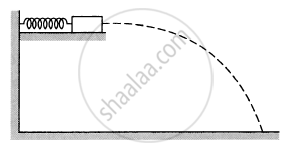Department of Pre-University Education, KarnatakaPUC Karnataka Science Class 11
Advertisement Remove all ads

# A small block of mass 100 g is pressed against a horizontal spring fixed at one end to compress the spring through 5 cm (figure following ). The spring constant is 100 N/m. - Physics

Numerical

A small block of mass 100 g is pressed against a horizontal spring fixed at one end to compress the spring through 5 cm (figure following). The spring constant is 100 N/m. When released, the block moves horizontally till it leaves the spring. Where will it hit the ground 2 m below the spring?Advertisement Remove all ads

#### Solution

$\text{Given}:$
$\text{ Mass of the block, m = 100 g = 0 . 1 kg }$
$\text{ Compression in the spring, x = 5 cm = 0 . 05 m }$
$\text{ Spring constant, k = 100 N/m }$

Let v be the velocity of the block when it leaves the spring.
Applying the law of conservation of energy,
Elastic potential energy of the spring = Kinetic energy of the block

$\frac{1}{2}\text{m} \nu^2 = \frac{1}{2}\text{kx}^2$

$\Rightarrow \nu = \text{x}\sqrt{\frac{\text{k}}{\text{m}}}$

$= \left( 0 . 05 \right) \times \sqrt{\frac{\left( 100 \right)}{0 . 1}}$

$= 1 . 58 \text{ m/s}$

For the projectile motion,

$\theta = 0^\circ, \text{ y } = - 2$
$\text{ Now, y }= \left( \text{ u } \cdot \sin \theta \right) \text{ t } - \frac{1}{2}\text{gt}^2$
$- 2 = \left( - \frac{1}{2} \right) \times \left( 9 . 8 \right) \times \text{t}^2$

$\Rightarrow \text{t = 0 . 63 \sec,}$
$\text{ So, x }= \left( \text{u \cos } \theta \right) \text{ t }$
$= \left( 1 . 58 \right) \times \left( 0 . 36 \right) = 1 \text{ m }$

Therefore, the block hits the ground at 1 m from the free end of the spring in the horizontal direction.

Is there an error in this question or solution?
Advertisement Remove all ads

#### APPEARS IN

HC Verma Class 11, 12 Concepts of Physics 1
Chapter 8 Work and Energy
Q 48 | Page 135
Advertisement Remove all ads
Advertisement Remove all ads
Share
Notifications

View all notifications

Forgot password?